Search:

Tudjon meg többet

Támogatás

Közreműködés

Fejlesztői státusz

# LGT (4/5) — Képlet szerkesztő

 To insert math use the menu Insert→Math→Inline Formula (shor-tcut Ctrl+m). This inserts a small blue box which will contain the formula. Note that the math toolbar pops up automatically if the cursor is in a math box.(Note also the footnote which was inserted using the menu Insert→Footnote.)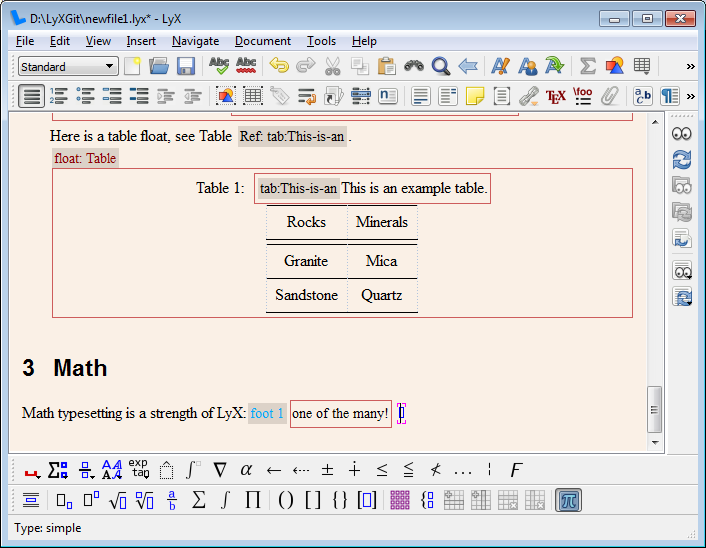Now start typing in the box. To get a superscript press "^ " (note the space; a subscript is "_") or the toolbar button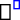. This adds a raised box to inform you that you are in a superscript.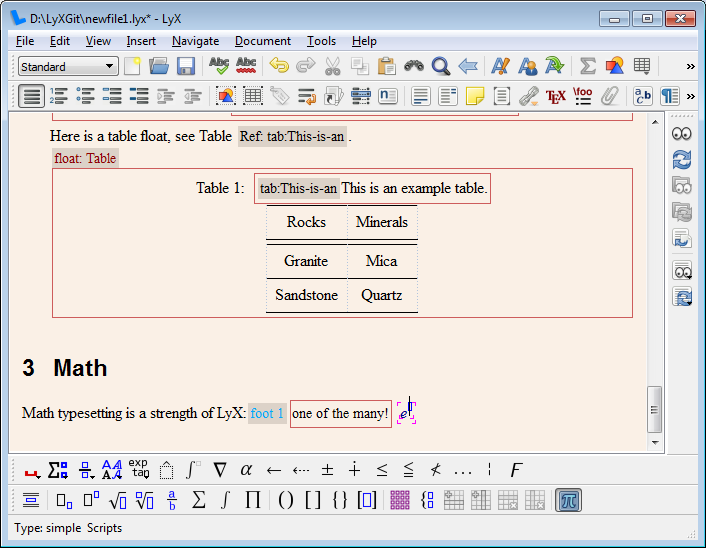To get a Greek letter (in this case the small pi) click in the math toolbar on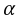and choose the pi. An often faster way is to type "\pi " (note the space) in the formula box.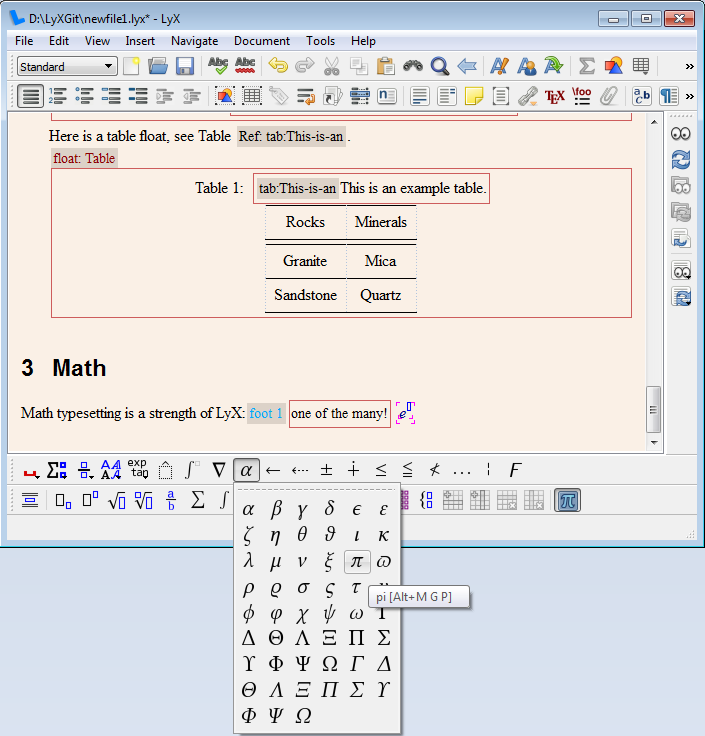The formula is basically ready.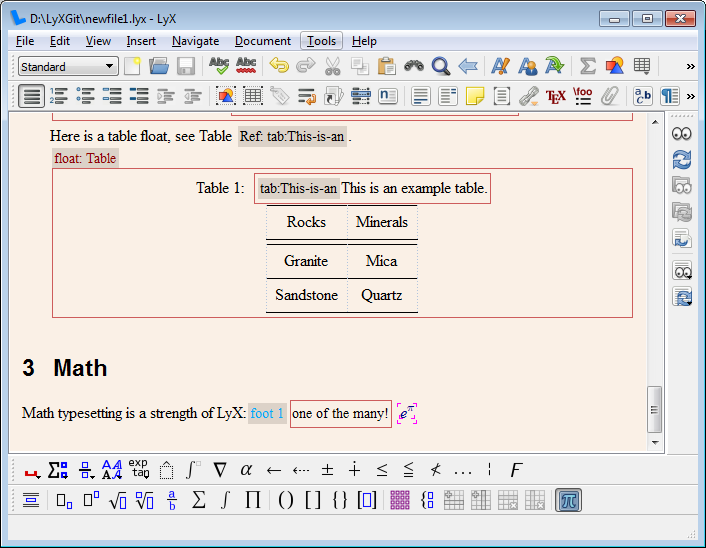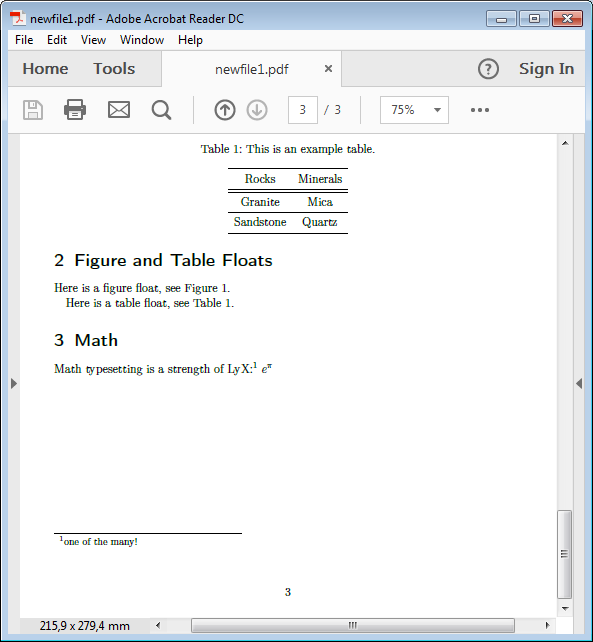To make it an equation that can also be referenced we need to change the formula tpye to displayed. This is done by going into the formula and clicking in the math toolbar on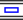(menu Edit→Math→Change Formula Type→Displayed Formula).A displayed formula can also be inserted directly via the Insert menu (shortcut Shift+Ctrl+m).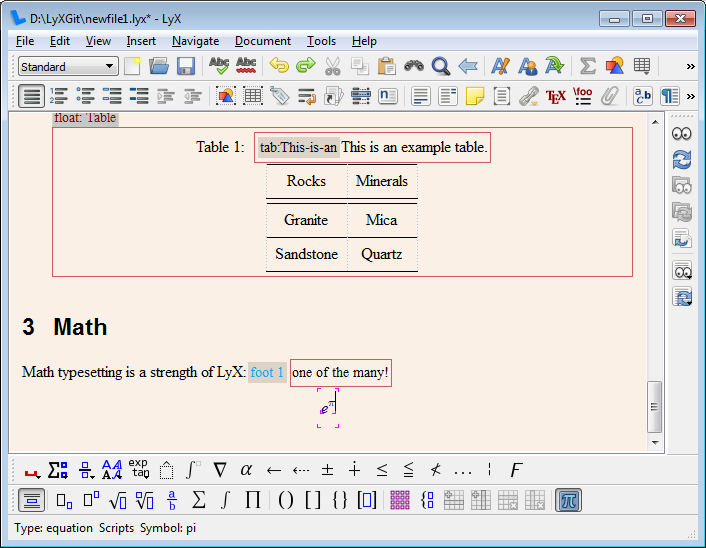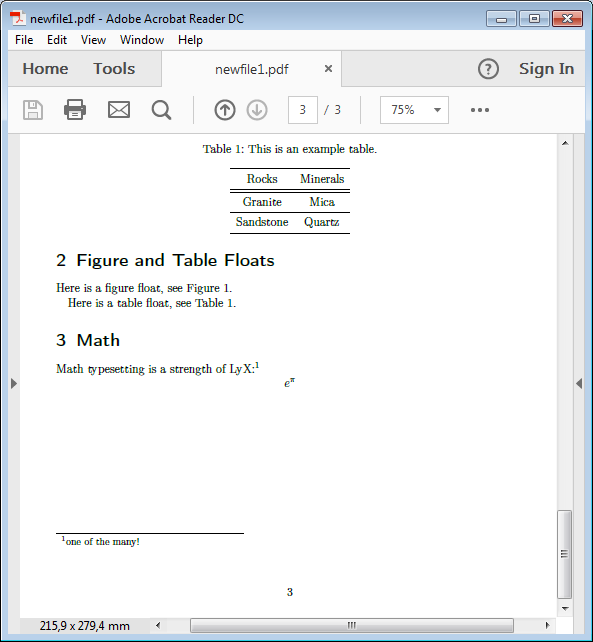Now we can add more to the formula to make it a real equation. To reference it, add a label. For formulas LyX will not propose a label name. You have to give one and you should keep the proposed prefix "eq:". The label is displayed in LyX behind the equation number.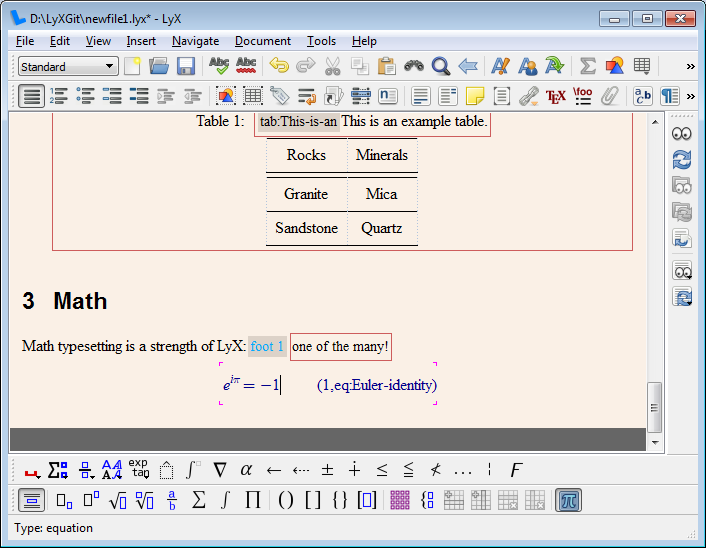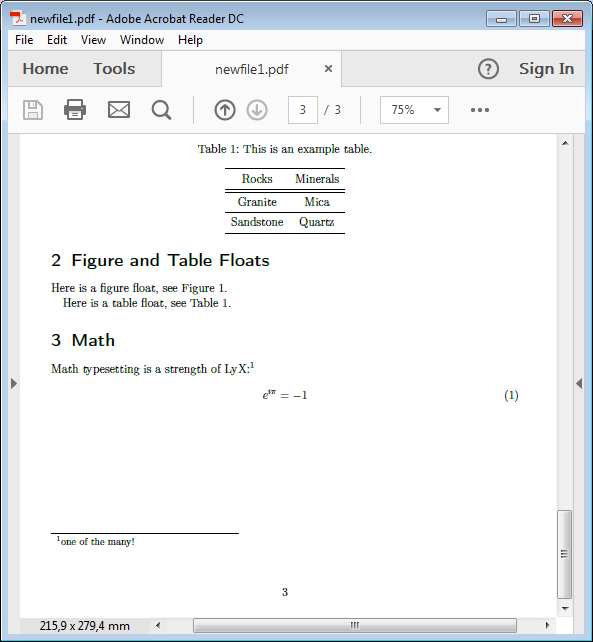The equation can now be referenced like tables and figures. The difference is that one usually adds round brackets around the reference number. They are added by clicking on the cross-reference. In the dialog change the reference format to ().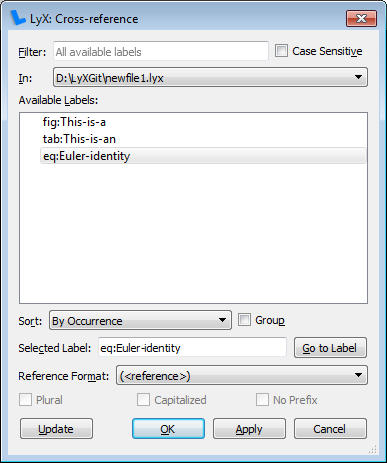Note that the reference box in LyX changed its prefix to "EqRef:" to indicate the format.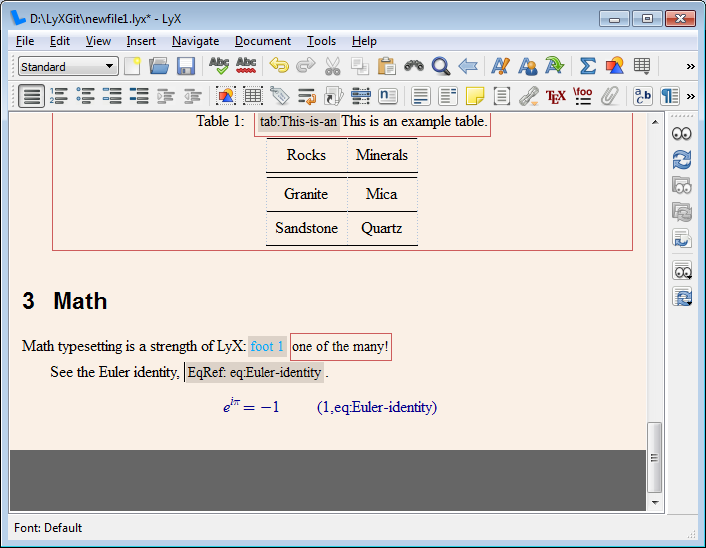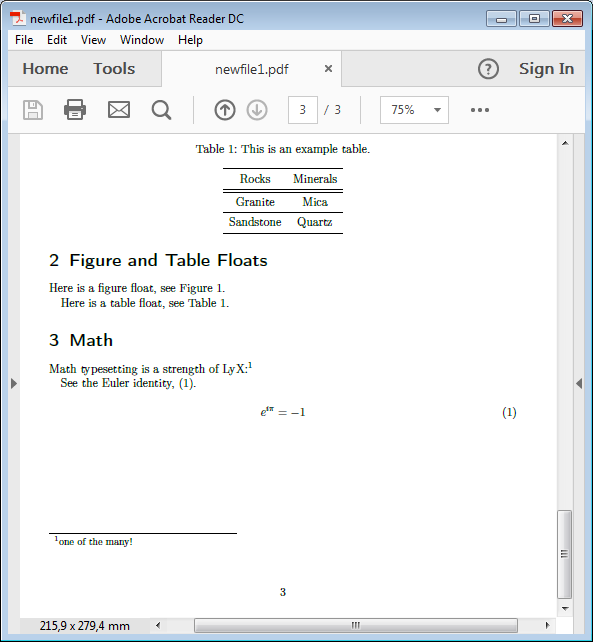By default variables are typeset italic. The imaginary unit 'i' should however be set upright to avoid mixing it up with other variables. This is done by highlighting the 'i' and using the math toolbar button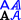. Select there Roman.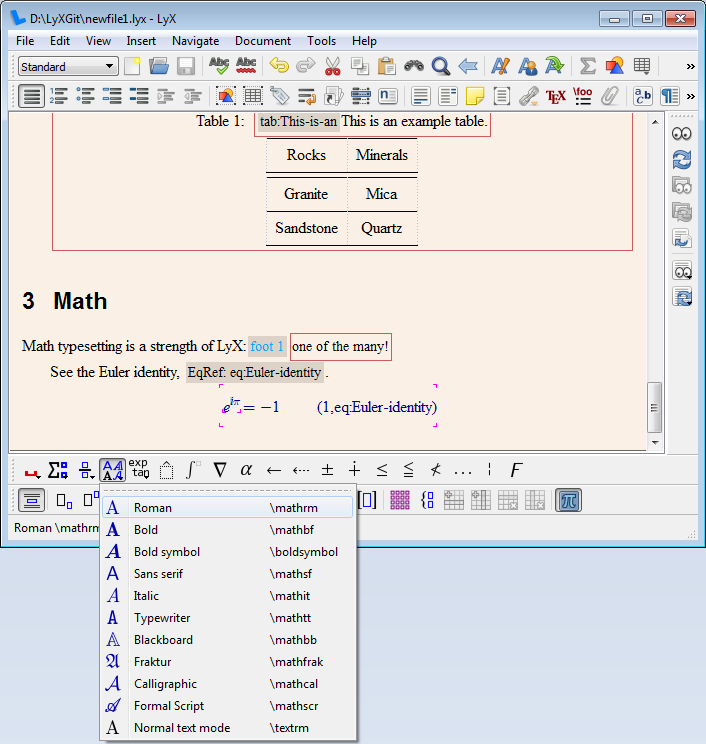We are now ready.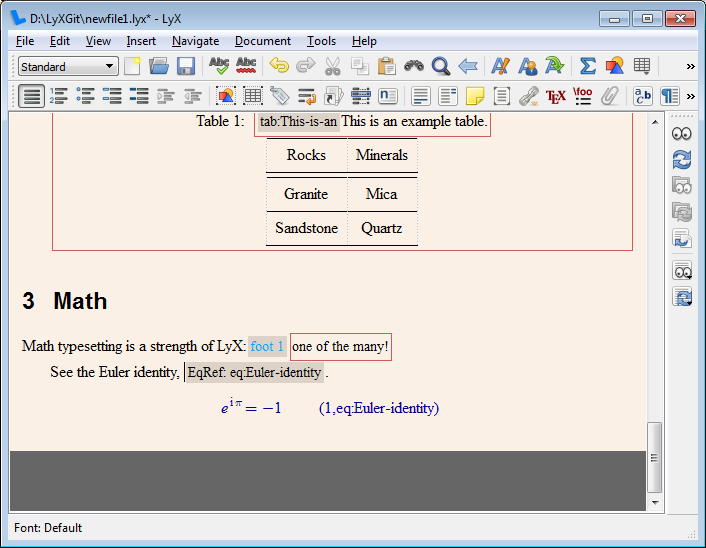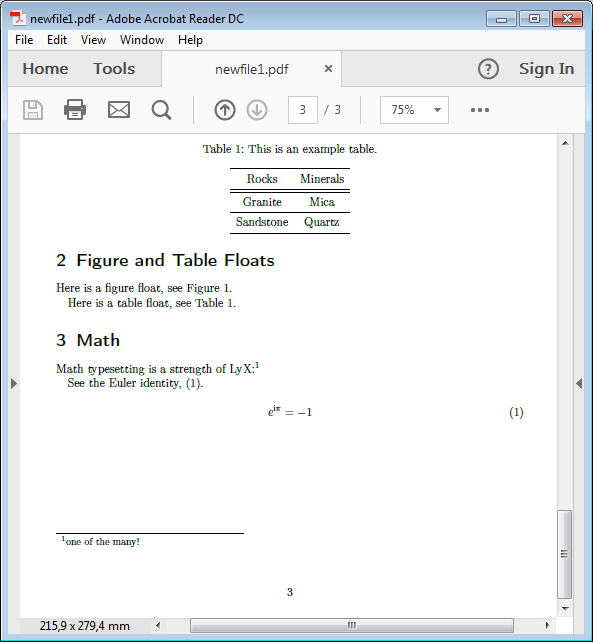-------------------- -------------------- --------------------